#Constrained Min/Max

This page is temporarily under construction

If you were hiking to the top of a hill, and looked out from the top, you would know that you were at a relative maximum of altitude. Indeed, if you had brought a coordinate system with you, or a compass to identify the East and North directions, you would see that if the altitude is represented as, then

###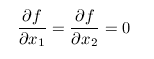at this point. You might also convince yourself that the second partials obey the condition for a maximum.

Suppose, however, that instead of going to the top, your path veers away from the summit, as in the contour map below. In his map, the dotted green curve is the path and the solid red curves are the contours of constant elevation.

##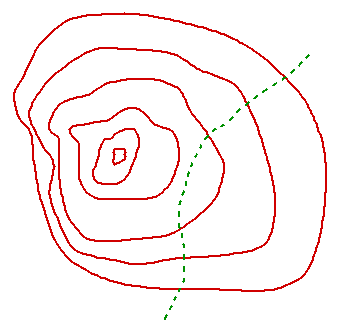Suppose that the green curve is described by

##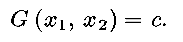Then the highest point reached while hiking along the path is the maximum of

##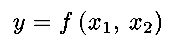subject to the constraint

##There are many ways to find this maximum height. One direct way is to assume that the path may be described by one variable as an explicit function of the other,

so that

##a function of a single variable. Then, differentiating and setting equal to zero, using the chain rule gives

Similarly, if

##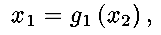then the condition for an extreme is

##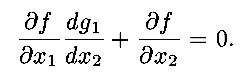Imagine again that you are hiking along the path as described above. At the maximum of your elevation, the steepest direction of the hillside would be to your sides, perpendicular to your path. That is, your path is perpendicular to the gradient of the function that describes the elevation, and tangent to the level sets (or contours) of the function. This is suggested in the figure above.

The path is a level set of the function G, and so the gradient of G must also be perpendicular to the path. That is, the gradients of f and G must be perpendicular,

##in the figure below, the arrow represents the common direction of the gradients.

##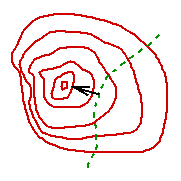But, if two vectors are parallel, their cross product must be zero, which in this case would mean that the condition for an extreme subject to the constraint is

If we note that

##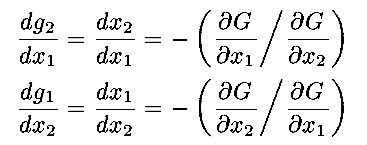we see that the above relations are recovered.

A generalization of the above method is shown in the next section, Lagrange Multipliers.

### Examples:

For both of these examples, the function whose extremes are to be found is the distance from a specified point. However, we recognize that maximizing the square of a distance maximizes the distance, and saves a good deal of work.

(1) Find the point on the line

##that is closest to the origin.

In this case,

so that

##may be found explicitly. Differentiating and setting equal to zero gives

##where the second coordinate is found from the constraint G=5.

The situtation is illustrated below; it can be seen that at the closest point, the line is tangent to a circle centered at the origin. (In this rigged problem, the tangent circle is the unit circle.)

##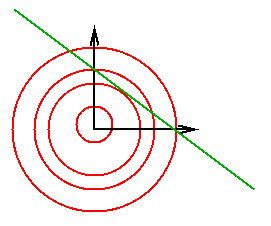(2) Find the point on the parabola

##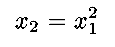that is closest to the point (0,a).

In this case,

and

##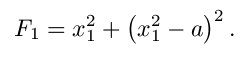(Note that finding one variable as an explicit function of the other is already done.) Differentiating and setting equal to zero gives

##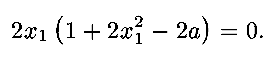In solving this, you must be careful: How many roots do you obtain, and why does the number of roots depend on the value of a? Are the solutions maxima or minima of the distance from the point? A figure (which you can draw yourself) might help.

Vector Calculus Index | World Web Math Main Page

watko@athena.mit.edu

Last modified February 10, 1999